# Chapter 7 RxODE events

## 7.1 RxODE event tables

In general, RxODE event tables follow NONMEM dataset convention with the exceptions:

• The compartment data item (`cmt`) can be a string/factor with compartment names
• You may turn off a compartment with a negative compartment number or “-cmt” where cmt is the compartment name.
• The compartment data item (`cmt`) can still be a number, the number of the compartment is defined by the appearance of the compartment name in the model. This can be tedious to count, so you can specify compartment numbers easier by using the `cmt(cmtName)` at the beginning of the model.
• An additional column, `dur` can specify the duration of infusions;
• Bioavailability changes will change the rate of infusion since `dur`/`amt` are fixed in the input data.
• Similarly, when specifying `rate`/`amt` for an infusion, the bioavailability will change the infusion duration since `rate`/`amt` are fixed in the input data.
• Some infrequent NONMEM columns are not supported: `pcmt`, `call`.
• `evid=5` or replace event; This replaces the value of a compartment with the value specified in the `amt` column. This is equivalent to `deSolve`=`replace`.
• `evid=6` or multiply event; This multiplies the value in the compartment with the value specified by the `amt` column. This is equivalent to `deSolve`=`multiply`.

Here are the legal entries to a data table:

Data Item Meaning Notes
id Individual identifier Can be a integer, factor, character, or numeric
time Individual time Numeric for each time.
amt dose amount Positive for doses zero/NA for observations
rate infusion rate When specified the infusion duration will be dur=amt/rate
rate = -1, rate modeled; rate = -2, duration modeled
dur infusion duration When specified the infusion rate will be rate = amt/dur
evid event ID 0=Observation; 1=Dose; 2=Other; 3=Reset; 4=Reset+Dose; 5=Replace; 6=Multiply
cmt Compartment Represents compartment #/name for dose/observation
ii Inter-dose Interval Time between doses.
addl # of additional doses Number of doses like the current dose.

Other notes:

• The `evid` can be the classic RxODE (described here) or the `NONMEM`-style `evid` described above.
• `NONMEM`’s `DV` is not required; `RxODE` is a ODE solving framework.
• `NONMEM`’s `MDV` is not required, since it is captured in `EVID`.
• Instead of `NONMEM`-compatible data, it can accept `deSolve` compatible data-frames.

When returning the RxODE solved data-set there are a few additional event ids (`EVID`) that you may see depending on the solving options:

• `EVID = -1` is when a modeled rate ends (corresponds to `rate = -1`)
• `EVID = -2` is when a modeled duration ends (corresponds to `rate=-2`)
• `EVID = -10` when a rate specified zero-order infusion ends (corresponds to `rate > 0`)
• `EVID = -20` when a duration specified zero-order infusion ends (corresponds to `dur > 0`)
• `EVID = 101, 102, 103,...` These correspond to the `1`, `2`, `3`, … modeled time (`mtime`).

These can only be accessed when solving with the option combination `addDosing=TRUE` and `subsetNonmem=FALSE`. If you want to see the classic `EVID` equivalents you can use `addDosing=NA`.

To illustrate the event types we will use the model from the original RxODE tutorial.

``````library(RxODE)
### Model from RxODE tutorial
m1 <-RxODE({
KA=2.94E-01;
CL=1.86E+01;
V2=4.02E+01;
Q=1.05E+01;
V3=2.97E+02;
Kin=1;
Kout=1;
EC50=200;
## Added modeled bioavaiblity, duration and rate
fdepot = 1;
durDepot = 8;
rateDepot = 1250;
C2 = centr/V2;
C3 = peri/V3;
d/dt(depot) =-KA*depot;
f(depot) = fdepot
dur(depot) = durDepot
rate(depot) = rateDepot
d/dt(centr) = KA*depot - CL*C2 - Q*C2 + Q*C3;
d/dt(peri)  =                    Q*C2 - Q*C3;
d/dt(eff)  = Kin - Kout*(1-C2/(EC50+C2))*eff;
eff(0) = 1
});``````

A bolus dose is the default type of dose in RxODE and only requires the `amt`/`dose`. Note that this uses the convenience function `et()` described in the RxODE event tables

``````ev <- et(timeUnits="hr") %>%
et(amt=10000, ii=12,until=24) %>%
et(seq(0, 24, length.out=100))

ev``````
``````#> ──────────────── EventTable with 101 records ───────────────
#>
#>    multiple doses in `addl` columns, expand with x\$expand(); or etExpand(x)
#> ── First part of x: ────────────────────────────────────────
#> # A tibble: 101 x 5
#>         time   amt    ii  addl evid
#>          [h] <dbl>   [h] <int> <evid>
#>  1 0.0000000    NA    NA    NA 0:Observation
#>  2 0.0000000 10000    12     2 1:Dose (Add)
#>  3 0.2424242    NA    NA    NA 0:Observation
#>  4 0.4848485    NA    NA    NA 0:Observation
#>  5 0.7272727    NA    NA    NA 0:Observation
#>  6 0.9696970    NA    NA    NA 0:Observation
#>  7 1.2121212    NA    NA    NA 0:Observation
#>  8 1.4545455    NA    NA    NA 0:Observation
#>  9 1.6969697    NA    NA    NA 0:Observation
#> 10 1.9393939    NA    NA    NA 0:Observation
#> # … with 91 more rows``````
``````rxSolve(m1, ev) %>% plot(C2) +
xlab("Time")``````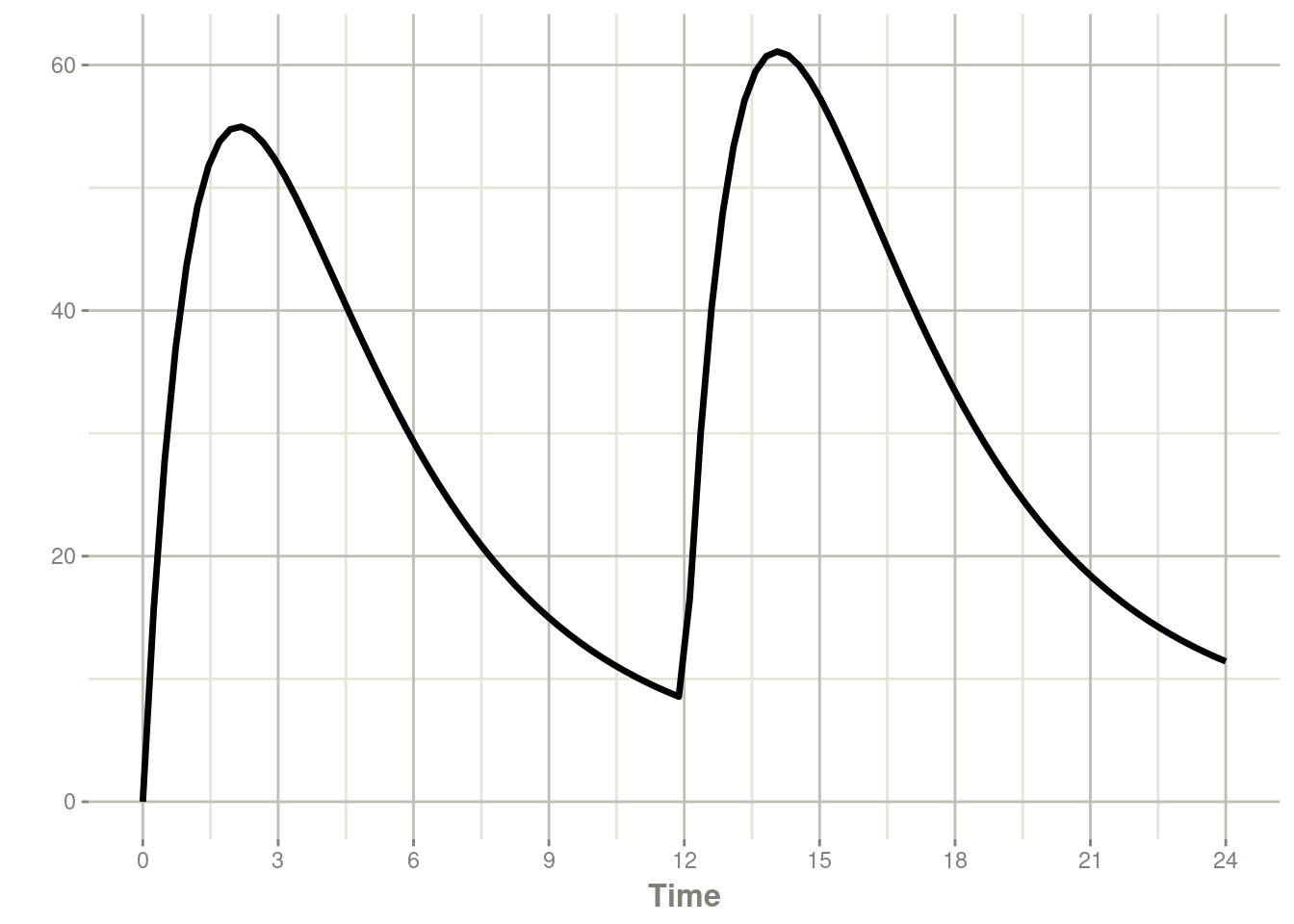## 7.3 Infusion Doses

There are a few different type of infusions that RxODE supports:

• Constant Rate Infusion (`rate`)
• Constant Duration Infusion (`dur`)
• Estimated Rate of Infusion
• Estimated Duration of Infusion

### 7.3.1 Constant Infusion (in terms of duration and rate)

The next type of event is an infusion; There are two ways to specify an infusion; The first is the `dur` keyword.

An example of this is:

``````ev <- et(timeUnits="hr") %>%
et(amt=10000, ii=12,until=24, dur=8) %>%
et(seq(0, 24, length.out=100))

ev``````
``````#> ──────────────── EventTable with 101 records ───────────────
#>
#>    multiple doses in `addl` columns, expand with x\$expand(); or etExpand(x)
#> ── First part of x: ────────────────────────────────────────
#> # A tibble: 101 x 6
#>         time   amt    ii  addl evid            dur
#>          [h] <dbl>   [h] <int> <evid>          [h]
#>  1 0.0000000    NA    NA    NA 0:Observation    NA
#>  2 0.0000000 10000    12     2 1:Dose (Add)      8
#>  3 0.2424242    NA    NA    NA 0:Observation    NA
#>  4 0.4848485    NA    NA    NA 0:Observation    NA
#>  5 0.7272727    NA    NA    NA 0:Observation    NA
#>  6 0.9696970    NA    NA    NA 0:Observation    NA
#>  7 1.2121212    NA    NA    NA 0:Observation    NA
#>  8 1.4545455    NA    NA    NA 0:Observation    NA
#>  9 1.6969697    NA    NA    NA 0:Observation    NA
#> 10 1.9393939    NA    NA    NA 0:Observation    NA
#> # … with 91 more rows``````
``````rxSolve(m1, ev) %>% plot(depot, C2) +
xlab("Time")``````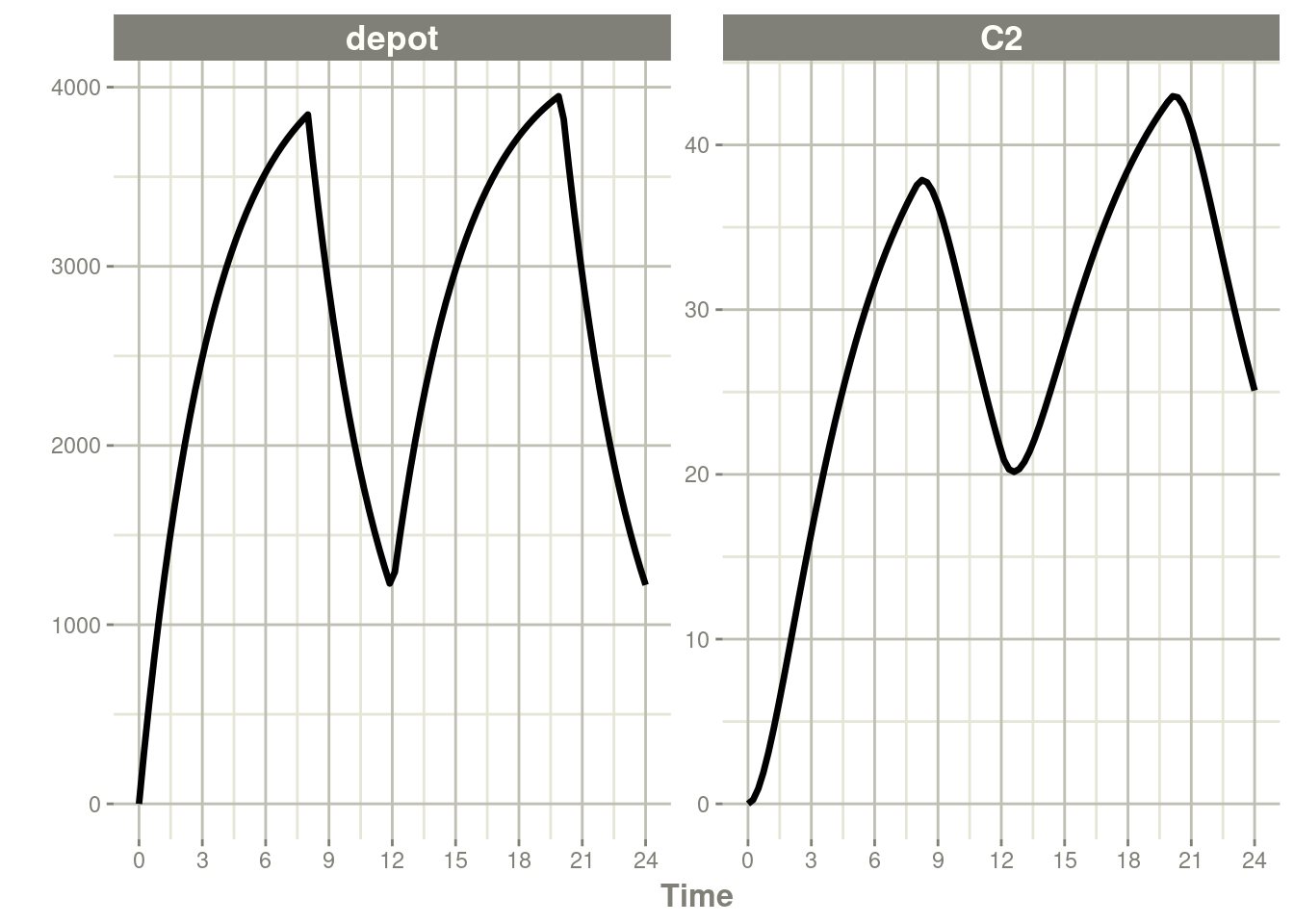It can be also specified by the `rate` component:

``````ev <- et(timeUnits="hr") %>%
et(amt=10000, ii=12,until=24, rate=10000/8) %>%
et(seq(0, 24, length.out=100))

ev``````
``````#> ──────────────── EventTable with 101 records ───────────────
#>
#>    multiple doses in `addl` columns, expand with x\$expand(); or etExpand(x)
#> ── First part of x: ────────────────────────────────────────
#> # A tibble: 101 x 6
#>         time   amt rate          ii  addl evid
#>          [h] <dbl> <rate/dur>   [h] <int> <evid>
#>  1 0.0000000    NA NA            NA    NA 0:Observation
#>  2 0.0000000 10000  1250         12     2 1:Dose (Add)
#>  3 0.2424242    NA NA            NA    NA 0:Observation
#>  4 0.4848485    NA NA            NA    NA 0:Observation
#>  5 0.7272727    NA NA            NA    NA 0:Observation
#>  6 0.9696970    NA NA            NA    NA 0:Observation
#>  7 1.2121212    NA NA            NA    NA 0:Observation
#>  8 1.4545455    NA NA            NA    NA 0:Observation
#>  9 1.6969697    NA NA            NA    NA 0:Observation
#> 10 1.9393939    NA NA            NA    NA 0:Observation
#> # … with 91 more rows``````
``````rxSolve(m1, ev) %>% plot(depot, C2) +
xlab("Time")``````These are the same with the exception of how bioavailability changes the infusion.

In the case of modeling `rate`, a bioavailability decrease, decreases the infusion duration, as in NONMEM. For example:

``````rxSolve(m1, ev, c(fdepot=0.25)) %>% plot(depot, C2) +
xlab("Time")``````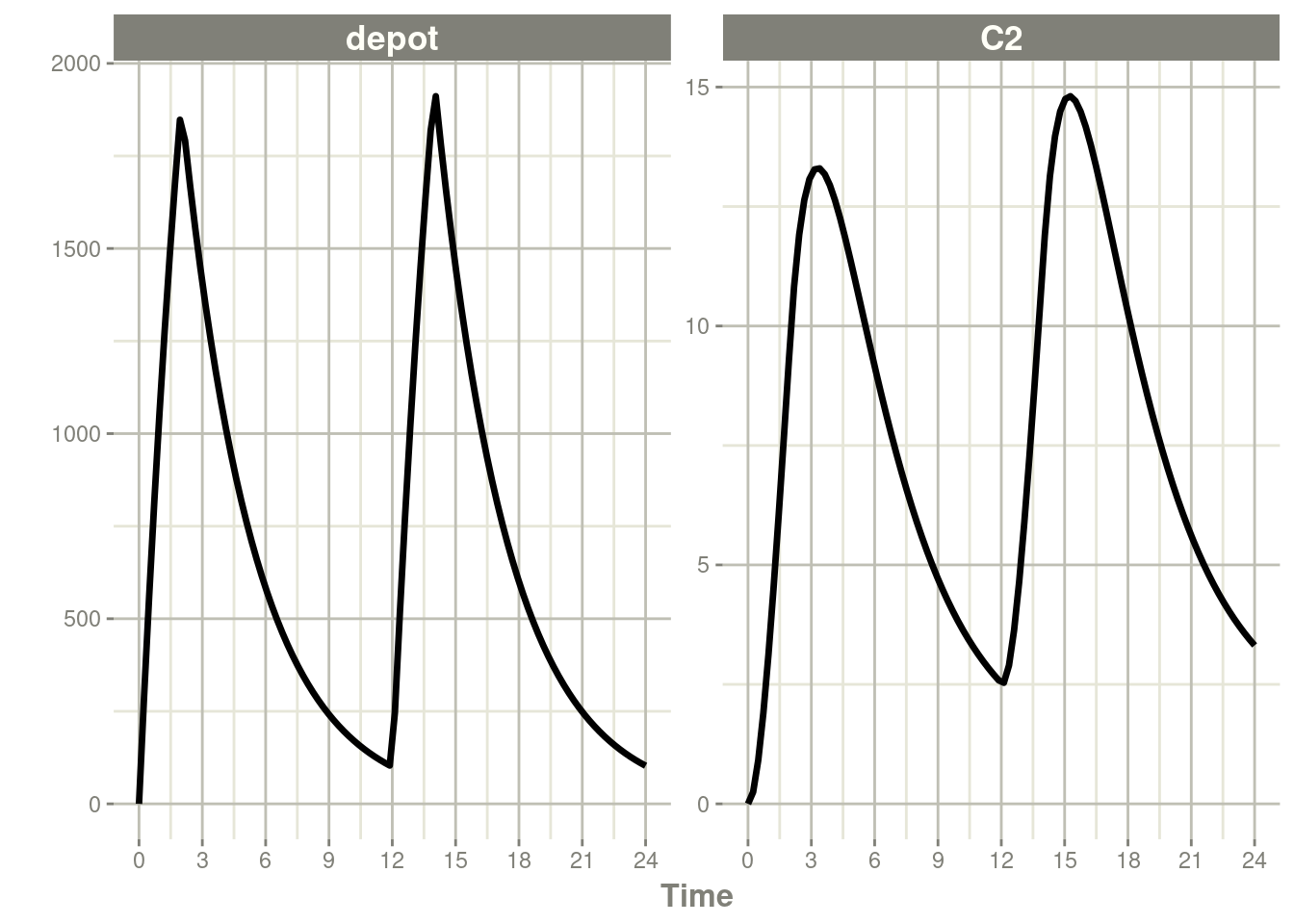Similarly increasing the bioavailability increases the infusion duration.

``````rxSolve(m1, ev, c(fdepot=1.25)) %>% plot(depot, C2) +
xlab("Time")``````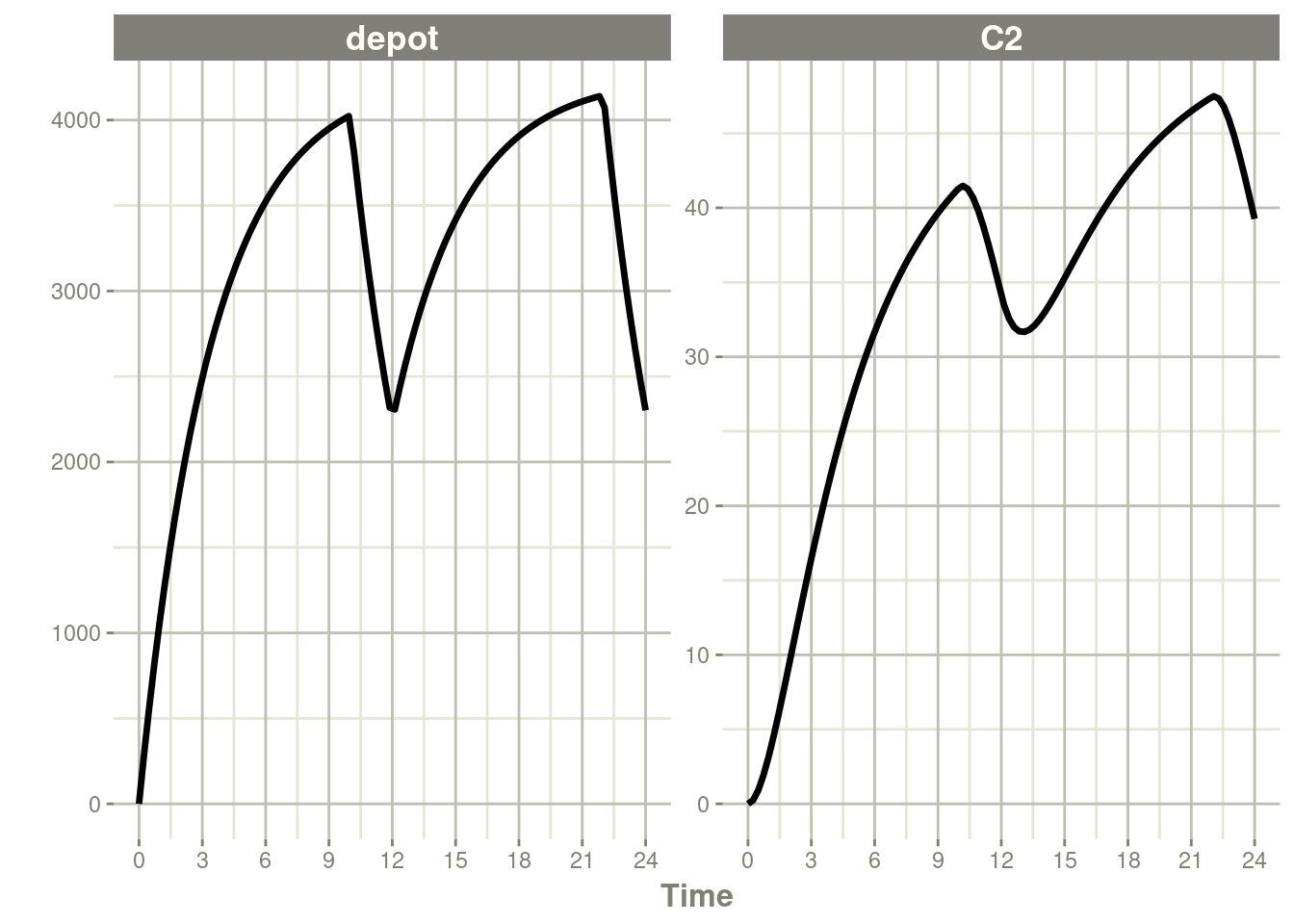The rationale for this behavior is that the `rate` and `amt` are specified by the event table, so the only thing that can change with a bioavailability increase is the duration of the infusion.

If you specify the `amt` and `dur` components in the event table, bioavailability changes affect the `rate` of infusion.

``````ev <- et(timeUnits="hr") %>%
et(amt=10000, ii=12,until=24, dur=8) %>%
et(seq(0, 24, length.out=100))``````

You can see the side-by-side comparison of bioavailability changes affecting `rate` instead of duration with these records in the following plots:

``````library(ggplot2)
library(patchwork)

p1 <- rxSolve(m1, ev, c(fdepot=1.25)) %>% plot(depot) +
xlab("Time") + ylim(0,5000)

p2 <- rxSolve(m1, ev, c(fdepot=0.25)) %>% plot(depot) +
xlab("Time")+ ylim(0,5000)

### Use patchwork syntax to combine plots
p1 * p2``````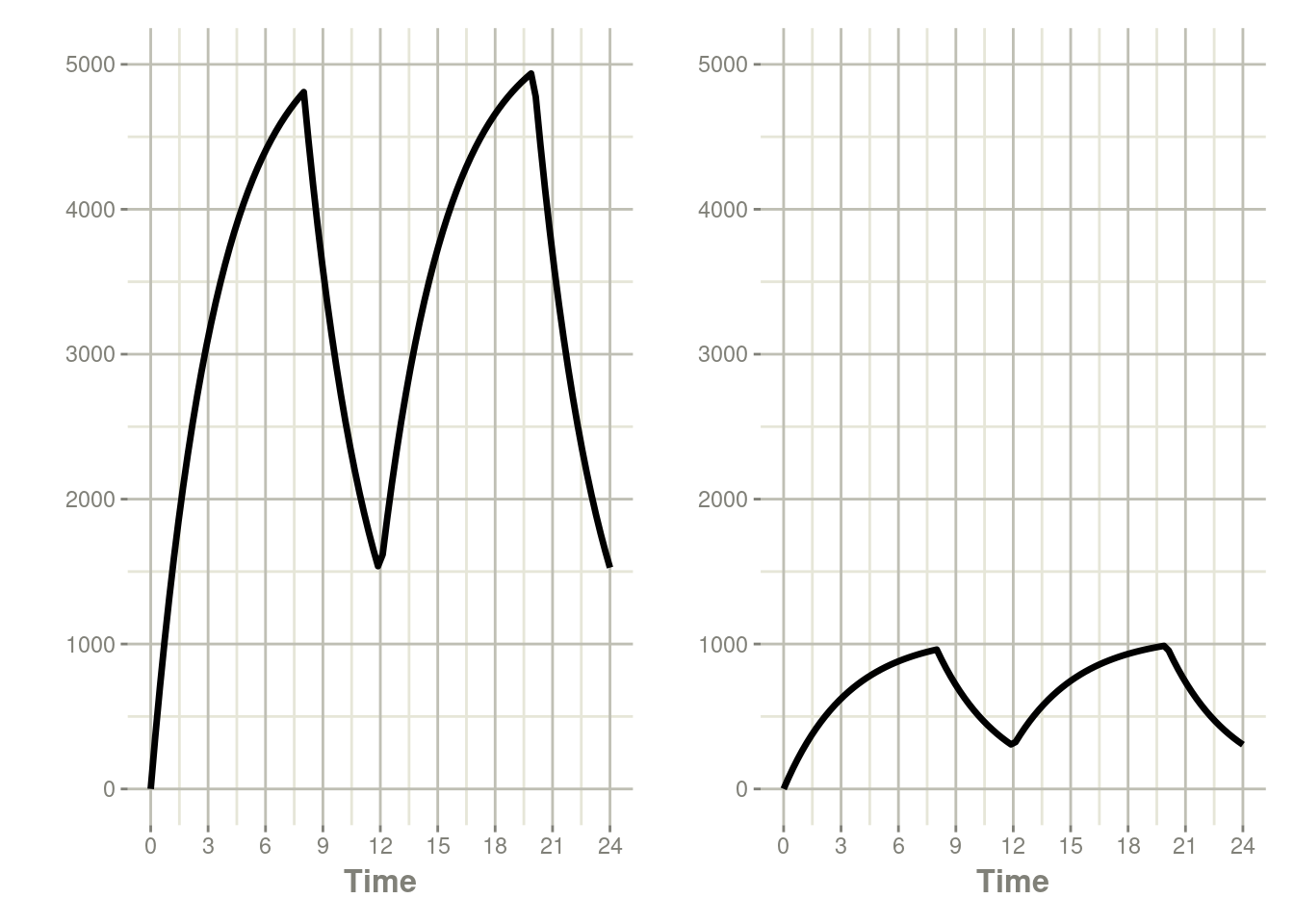### 7.3.2 Modeled Rate and Duration of Infusion

You can model the duration, which is equivalent to NONMEM’s `rate=-2`. As a mnemonic you can use the `dur=model` instead of `rate=-2`

``````ev <- et(timeUnits="hr") %>%
et(amt=10000, ii=12,until=24, dur=model) %>%
et(seq(0, 24, length.out=100))

ev``````
``````#> ──────────────── EventTable with 101 records ───────────────
#>
#>    multiple doses in `addl` columns, expand with x\$expand(); or etExpand(x)
#> ── First part of x: ────────────────────────────────────────
#> # A tibble: 101 x 6
#>         time   amt rate          ii  addl evid
#>          [h] <dbl> <rate/dur>   [h] <int> <evid>
#>  1 0.0000000    NA NA            NA    NA 0:Observation
#>  2 0.0000000 10000 -2:dur        12     2 1:Dose (Add)
#>  3 0.2424242    NA NA            NA    NA 0:Observation
#>  4 0.4848485    NA NA            NA    NA 0:Observation
#>  5 0.7272727    NA NA            NA    NA 0:Observation
#>  6 0.9696970    NA NA            NA    NA 0:Observation
#>  7 1.2121212    NA NA            NA    NA 0:Observation
#>  8 1.4545455    NA NA            NA    NA 0:Observation
#>  9 1.6969697    NA NA            NA    NA 0:Observation
#> 10 1.9393939    NA NA            NA    NA 0:Observation
#> # … with 91 more rows``````
``````rxSolve(m1, ev, c(durDepot=7)) %>% plot(depot, C2) +
xlab("Time")``````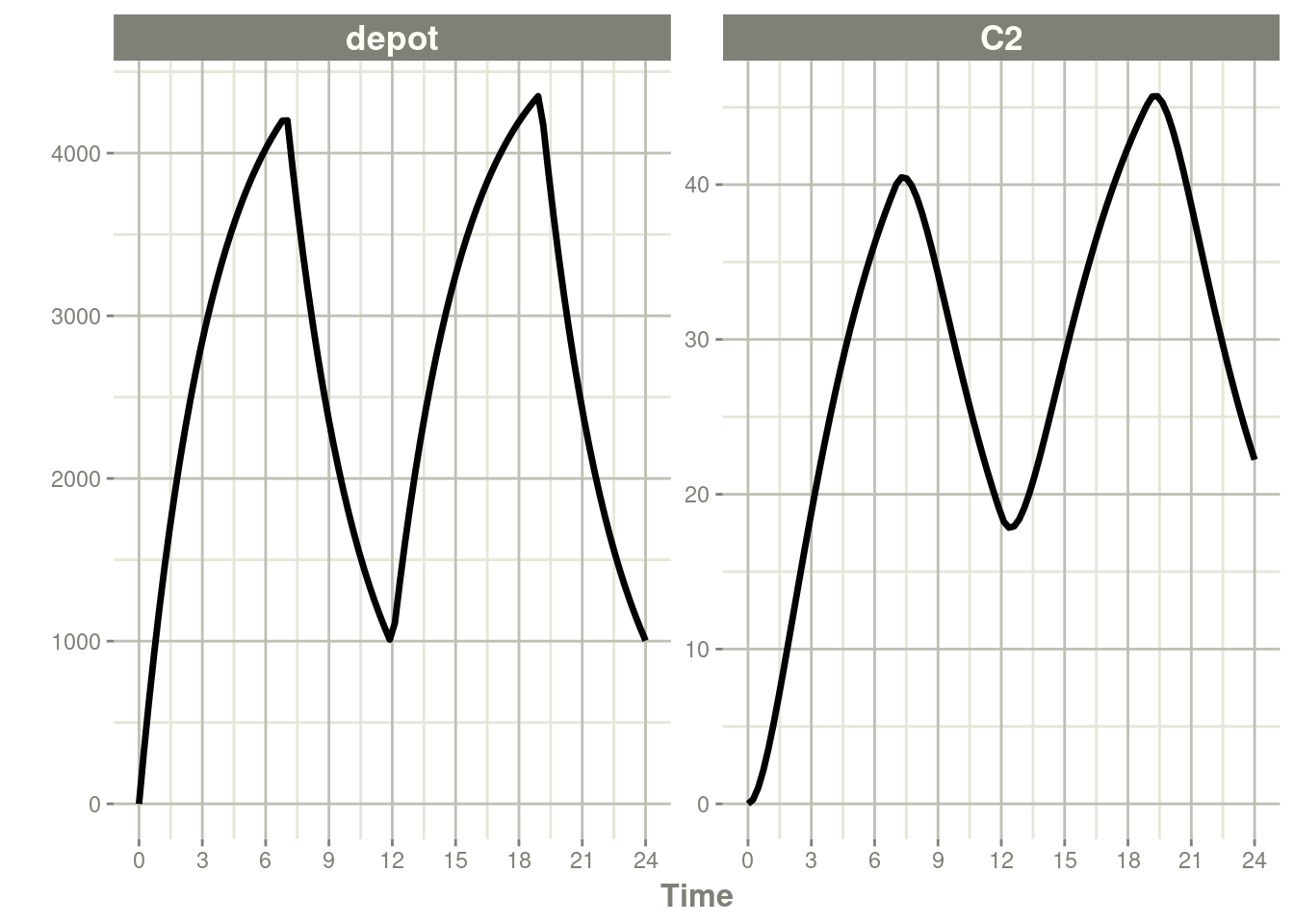Similarly, you may also model rate. This is equivalent to NONMEM’s `rate=-1` and is how RxODE’s event table specifies the data item as well. You can also use `rate=model` as a mnemonic:

``````ev <- et(timeUnits="hr") %>%
et(amt=10000, ii=12,until=24, rate=model) %>%
et(seq(0, 24, length.out=100))

ev``````
``````#> ──────────────── EventTable with 101 records ───────────────
#>
#>    multiple doses in `addl` columns, expand with x\$expand(); or etExpand(x)
#> ── First part of x: ────────────────────────────────────────
#> # A tibble: 101 x 6
#>         time   amt rate          ii  addl evid
#>          [h] <dbl> <rate/dur>   [h] <int> <evid>
#>  1 0.0000000    NA NA            NA    NA 0:Observation
#>  2 0.0000000 10000 -1:rate       12     2 1:Dose (Add)
#>  3 0.2424242    NA NA            NA    NA 0:Observation
#>  4 0.4848485    NA NA            NA    NA 0:Observation
#>  5 0.7272727    NA NA            NA    NA 0:Observation
#>  6 0.9696970    NA NA            NA    NA 0:Observation
#>  7 1.2121212    NA NA            NA    NA 0:Observation
#>  8 1.4545455    NA NA            NA    NA 0:Observation
#>  9 1.6969697    NA NA            NA    NA 0:Observation
#> 10 1.9393939    NA NA            NA    NA 0:Observation
#> # … with 91 more rows``````
``````rxSolve(m1, ev, c(rateDepot=10000/3)) %>% plot(depot, C2) +
xlab("Time")``````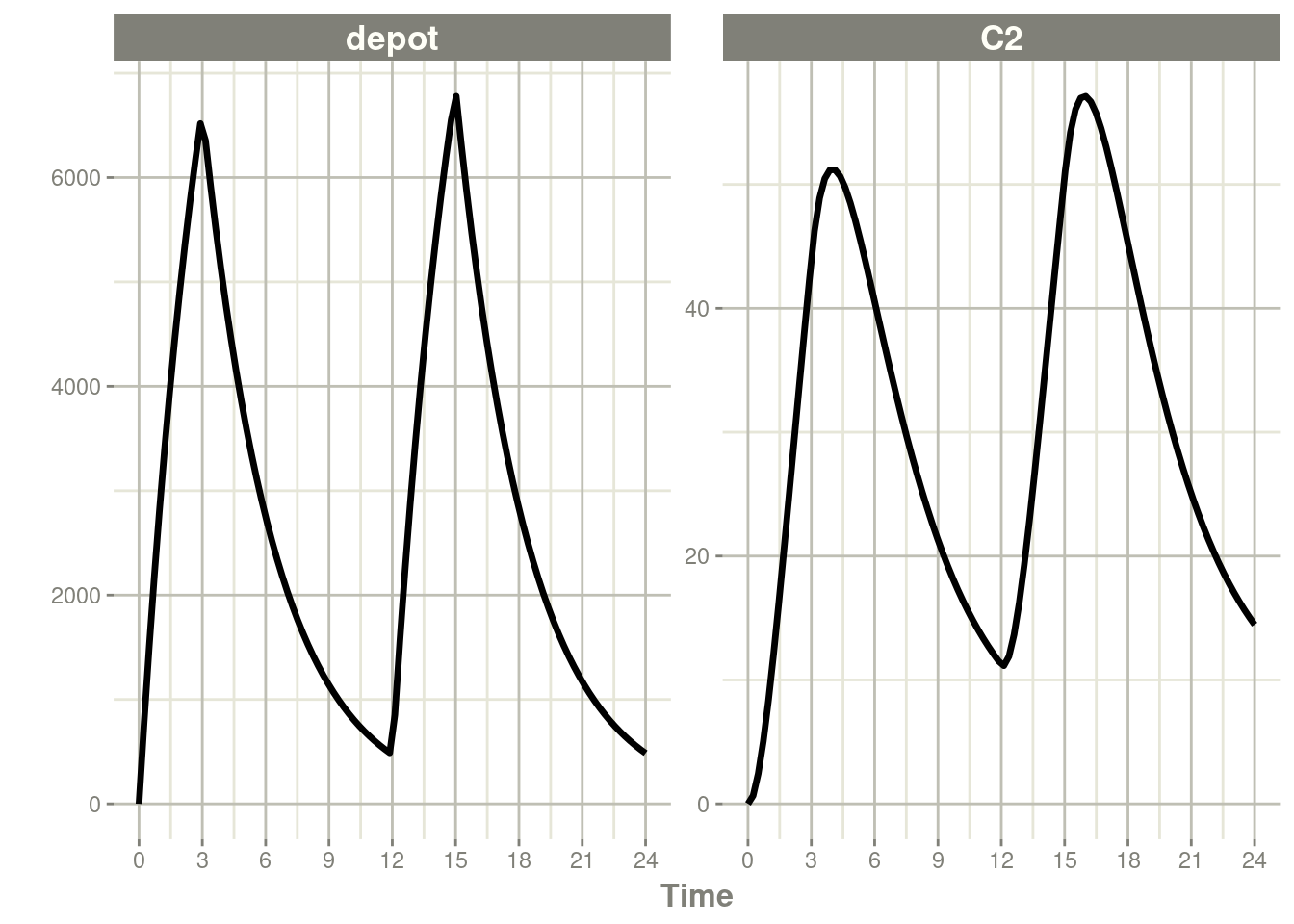These doses are solved until a steady state is reached with a constant inter-dose interval.

``````ev <- et(timeUnits="hr") %>%
et(amt=10000, ii=12, ss=1) %>%
et(seq(0, 24, length.out=100))

ev``````
``````#> ──────────────── EventTable with 101 records ───────────────
#>
#> ── First part of x: ────────────────────────────────────────
#> # A tibble: 101 x 5
#>         time   amt    ii evid             ss
#>          [h] <dbl>   [h] <evid>        <int>
#>  1 0.0000000    NA    NA 0:Observation    NA
#>  2 0.0000000 10000    12 1:Dose (Add)      1
#>  3 0.2424242    NA    NA 0:Observation    NA
#>  4 0.4848485    NA    NA 0:Observation    NA
#>  5 0.7272727    NA    NA 0:Observation    NA
#>  6 0.9696970    NA    NA 0:Observation    NA
#>  7 1.2121212    NA    NA 0:Observation    NA
#>  8 1.4545455    NA    NA 0:Observation    NA
#>  9 1.6969697    NA    NA 0:Observation    NA
#> 10 1.9393939    NA    NA 0:Observation    NA
#> # … with 91 more rows``````
``rxSolve(m1, ev) %>% plot(C2)``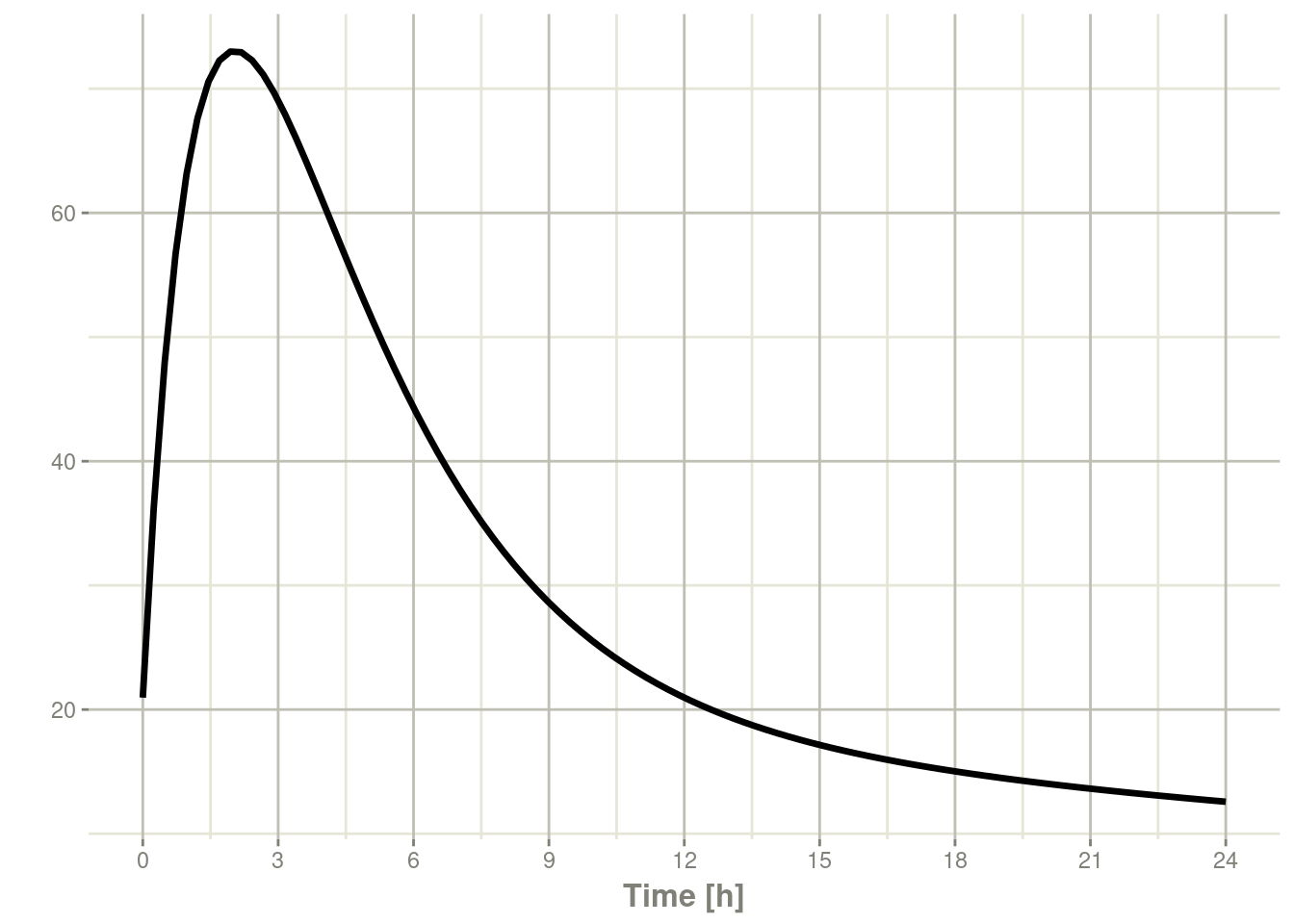### 7.4.1 Steady state for complex dosing

By using the `ss=2` flag, you can use the super-positioning principle in linear kinetics to get steady state nonstandard dosing (i.e. morning 100 mg vs evening 150 mg). This is done by:

• Saving all the state values
• Resetting all the states and solving the system to steady state
• Adding back all the prior state values
``````ev <- et(timeUnits="hr") %>%
et(amt=10000, ii=24, ss=1) %>%
et(time=12, amt=15000, ii=24, ss=2) %>%
et(seq(0, 64, length.out=500))

library(ggplot2)

rxSolve(m1, ev,maxsteps=10000) %>% plot(C2) +
annotate("rect", xmin=0, xmax=24, ymin=-Inf, ymax=Inf,
alpha=0.2) +
annotate("text", x=12.5, y=7,
annotate("text", x=44,   y=7,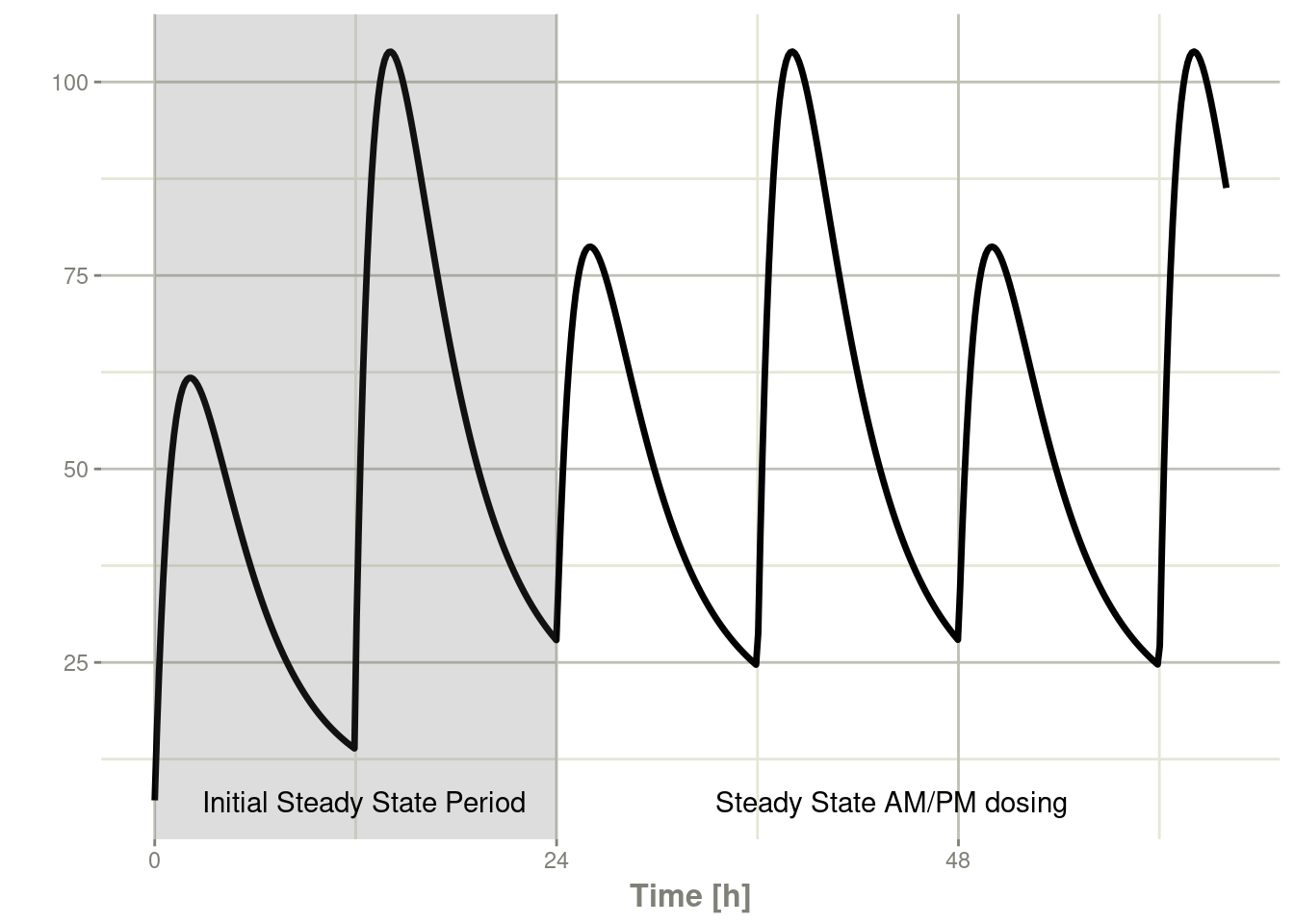You can see that it takes a full dose cycle to reach the true complex steady state dosing.

### 7.4.2 Steady state for constant infusion or zero order processes

The last type of steady state that RxODE supports is steady-state constant infusion rate. This can be specified the same way as NONMEM, that is:

• No inter-dose interval `ii`=`0`
• A steady state dose, ie `ss`=`1`
• Either a positive rate (`rate`>0) or a estimated rate `rate`=`-1`.
• A zero dose, ie `amt`=`0`
• Once the steady-state constant infusion is achieved, the infusion is turned off when using this record, just like NONMEM.

Note that `rate`=`-2` where we model the duration of infusion doesn’t make much sense since we are solving the infusion until steady state. The duration is specified by the steady state solution.

Also note that bioavailability changes on this steady state infusion also do not make sense because they neither change the `rate` or the duration of the steady state infusion. Hence modeled bioavailability on this type of dosing event is ignored.

Here is an example:

``````ev <- et(timeUnits="hr") %>%
et(amt=0, ss=1,rate=10000/8)

p1 <- rxSolve(m1, ev) %>% plot(C2, eff)

ev <- et(timeUnits="hr") %>%
et(amt=200000, rate=10000/8) %>%
et(0, 250, length.out=1000)

p2 <- rxSolve(m1, ev) %>% plot(C2, eff)

library(patchwork)

p1 / p2``````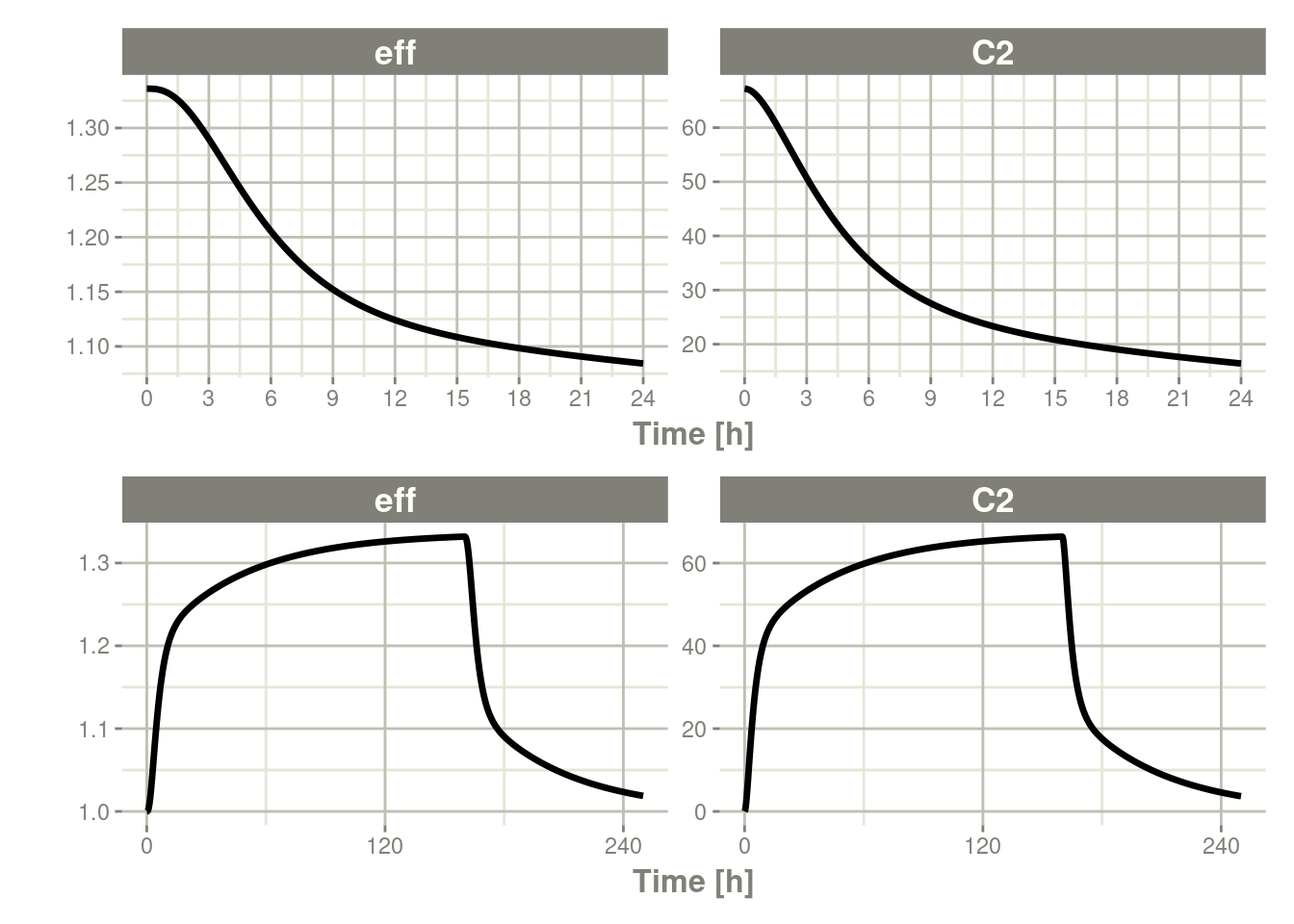Not only can this be used for PK, it can be used for steady-state disease processes.

## 7.5 Reset Events

Reset events are implemented by `evid=3` or `evid=reset`, for reset and `evid=4` for reset and dose.

``````ev <- et(timeUnits="hr") %>%
et(time=6, evid=reset) %>%
et(seq(0, 24, length.out=100))

ev``````
``````#> ──────────────── EventTable with 102 records ───────────────
#>
#>    multiple doses in `addl` columns, expand with x\$expand(); or etExpand(x)
#> ── First part of x: ────────────────────────────────────────
#> # A tibble: 102 x 5
#>         time   amt    ii  addl evid
#>          [h] <dbl>   [h] <int> <evid>
#>  1 0.0000000    NA    NA    NA 0:Observation
#>  2 0.0000000 10000    12     3 1:Dose (Add)
#>  3 0.2424242    NA    NA    NA 0:Observation
#>  4 0.4848485    NA    NA    NA 0:Observation
#>  5 0.7272727    NA    NA    NA 0:Observation
#>  6 0.9696970    NA    NA    NA 0:Observation
#>  7 1.2121212    NA    NA    NA 0:Observation
#>  8 1.4545455    NA    NA    NA 0:Observation
#>  9 1.6969697    NA    NA    NA 0:Observation
#> 10 1.9393939    NA    NA    NA 0:Observation
#> # … with 92 more rows``````

The solving show what happens in this system when the system is reset at 6 hours post-dose.

``rxSolve(m1, ev) %>% plot(depot,C2, eff)``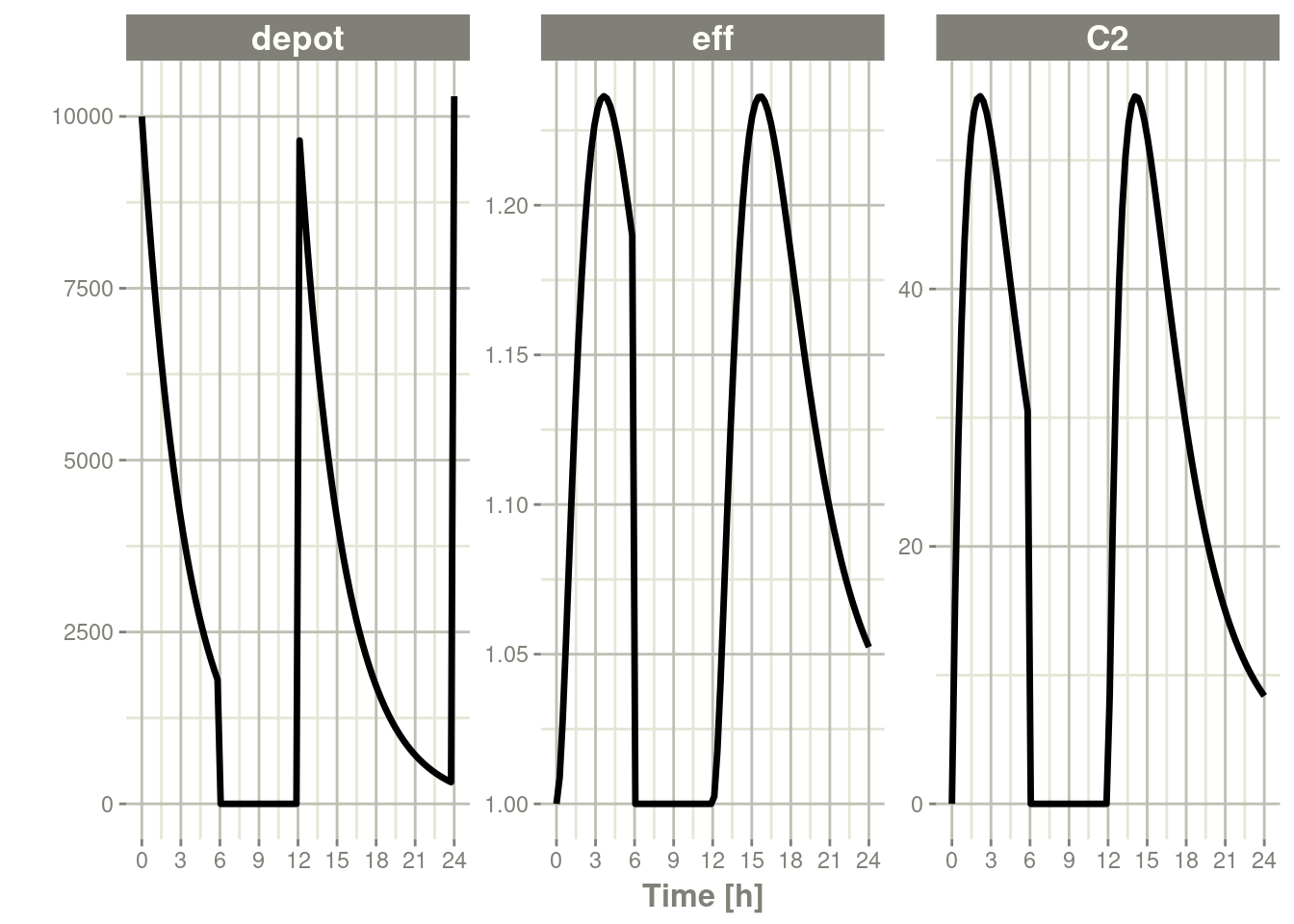You can see all the compartments are reset to their initial values. The next dose start the dosing cycle over.

``````ev <- et(timeUnits="hr") %>%
et(time=6, amt=10000, evid=4) %>%
et(seq(0, 24, length.out=100))

ev``````
``````#> ──────────────── EventTable with 102 records ───────────────
#>
#>    multiple doses in `addl` columns, expand with x\$expand(); or etExpand(x)
#> ── First part of x: ────────────────────────────────────────
#> # A tibble: 102 x 5
#>         time   amt    ii  addl evid
#>          [h] <dbl>   [h] <int> <evid>
#>  1 0.0000000    NA    NA    NA 0:Observation
#>  2 0.0000000 10000    12     3 1:Dose (Add)
#>  3 0.2424242    NA    NA    NA 0:Observation
#>  4 0.4848485    NA    NA    NA 0:Observation
#>  5 0.7272727    NA    NA    NA 0:Observation
#>  6 0.9696970    NA    NA    NA 0:Observation
#>  7 1.2121212    NA    NA    NA 0:Observation
#>  8 1.4545455    NA    NA    NA 0:Observation
#>  9 1.6969697    NA    NA    NA 0:Observation
#> 10 1.9393939    NA    NA    NA 0:Observation
#> # … with 92 more rows``````

In this case, the whole system is reset and the dose is given

``rxSolve(m1, ev) %>% plot(depot,C2, eff)``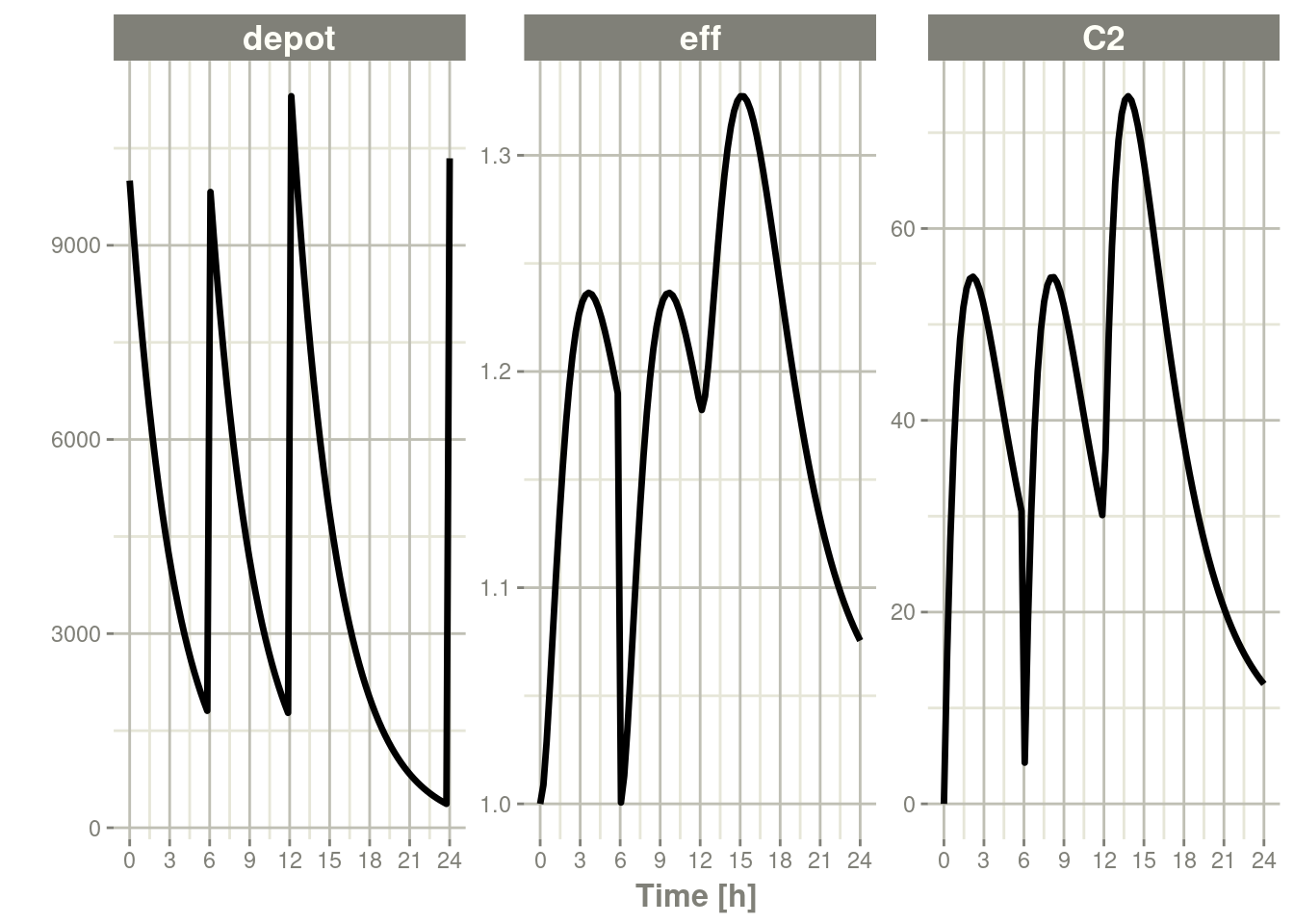## 7.6 Turning off compartments

You may also turn off a compartment, which is similar to a reset event.

``````ev <- et(timeUnits="hr") %>%
et(time=6, cmt="-depot", evid=2) %>%
et(seq(0, 24, length.out=100))

ev``````
``````#> ──────────────── EventTable with 102 records ───────────────
#>
#>    multiple doses in `addl` columns, expand with x\$expand(); or etExpand(x)
#> ── First part of x: ────────────────────────────────────────
#> # A tibble: 102 x 6
#>         time cmt         amt    ii  addl evid
#>          [h] <chr>     <dbl>   [h] <int> <evid>
#>  1 0.0000000 (obs)        NA    NA    NA 0:Observation
#>  2 0.0000000 (default) 10000    12     3 1:Dose (Add)
#>  3 0.2424242 (obs)        NA    NA    NA 0:Observation
#>  4 0.4848485 (obs)        NA    NA    NA 0:Observation
#>  5 0.7272727 (obs)        NA    NA    NA 0:Observation
#>  6 0.9696970 (obs)        NA    NA    NA 0:Observation
#>  7 1.2121212 (obs)        NA    NA    NA 0:Observation
#>  8 1.4545455 (obs)        NA    NA    NA 0:Observation
#>  9 1.6969697 (obs)        NA    NA    NA 0:Observation
#> 10 1.9393939 (obs)        NA    NA    NA 0:Observation
#> # … with 92 more rows``````

Solving shows what this does in the system:

``rxSolve(m1, ev) %>% plot(depot,C2, eff)``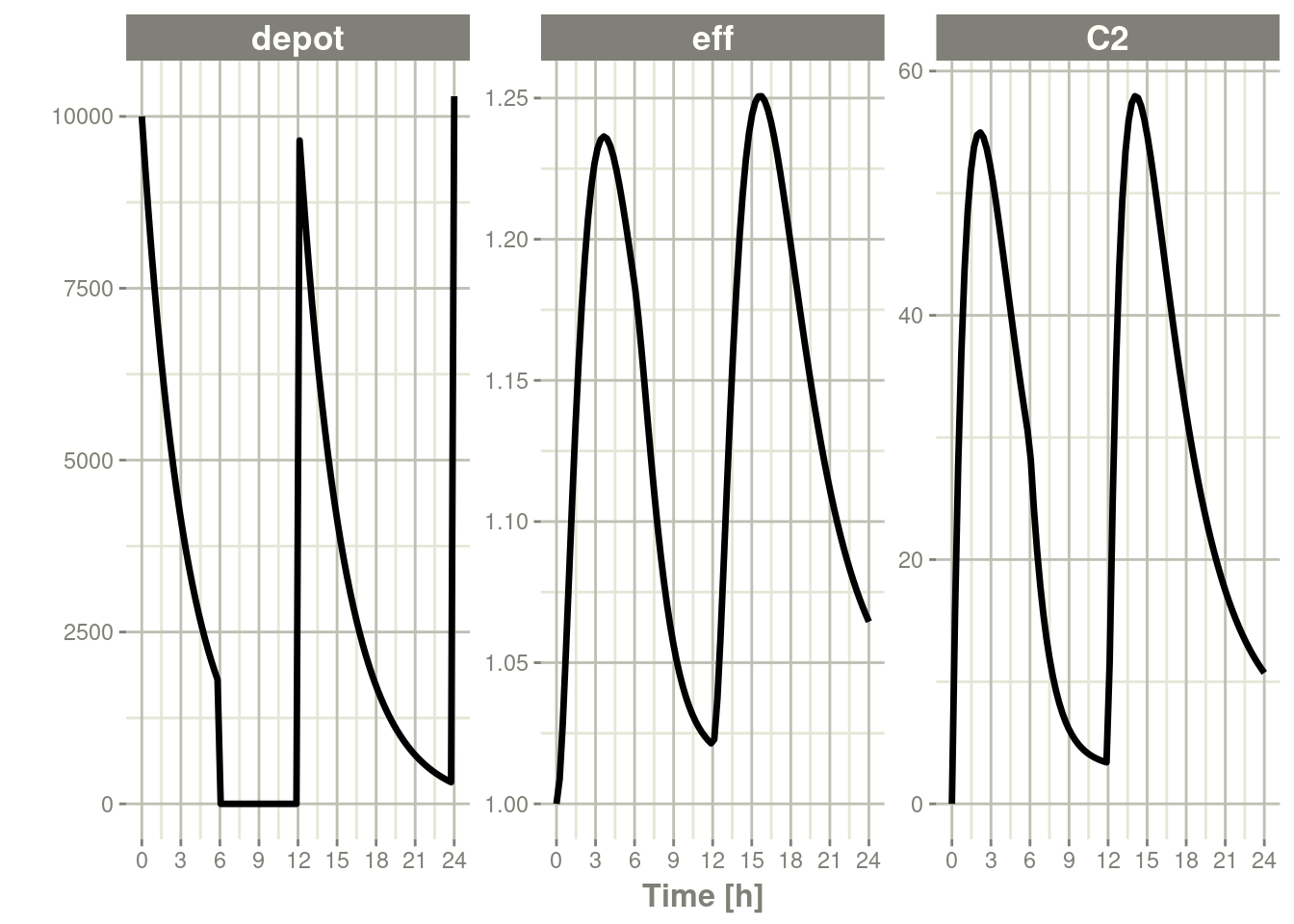In this case, the depot is turned off, and the depot compartment concentrations are set to the initial values but the other compartment concentrations/levels are not reset. When another dose to the depot is administered the depot compartment is turned back on.

Note that a dose to a compartment only turns back on the compartment that was dosed. Hence if you turn off the effect compartment, it continues to be off after another dose to the depot.

``````ev <- et(timeUnits="hr") %>%
et(time=6, cmt="-eff", evid=2) %>%
et(seq(0, 24, length.out=100))

rxSolve(m1, ev) %>% plot(depot,C2, eff)``````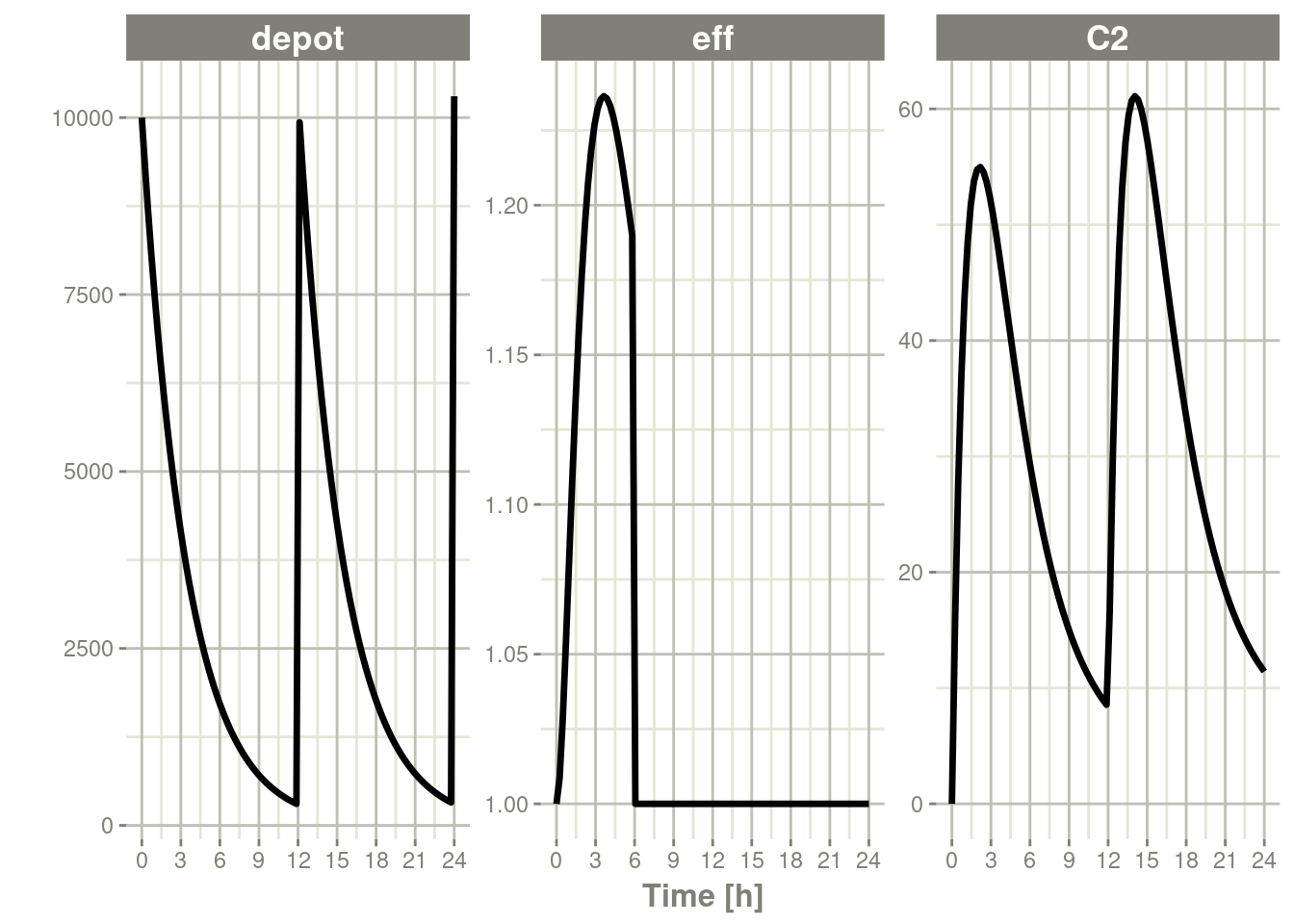To turn back on the compartment, a zero-dose to the compartment or a evid=2 with the compartment would be needed.

``````ev <- et(timeUnits="hr") %>%
et(time=6, cmt="-eff", evid=2) %>%
et(time=12,cmt="eff",evid=2) %>%
et(seq(0, 24, length.out=100))

rxSolve(m1, ev) %>% plot(depot,C2, eff)``````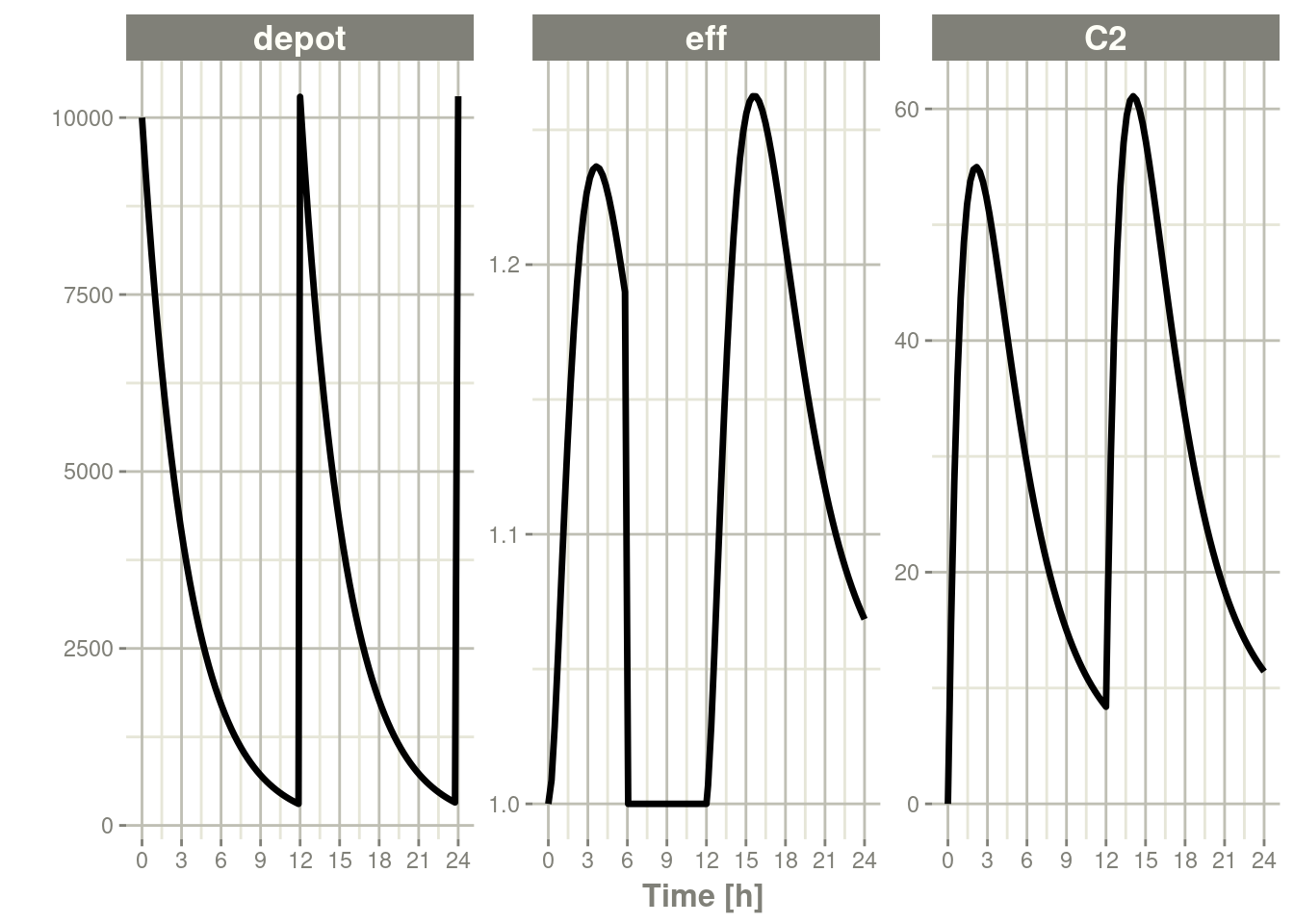## 7.7 Classic RxODE events

Originally RxODE supported compound event IDs; RxODE still supports these parameters, but it is often more useful to use the the normal NONMEM dataset standard that is used by many modeling tools like NONMEM, Monolix and nlmixr, described in the RxODE event types article.

Classically, RxODE supported event coding in a single event id `evid` described in the following table.

100+ cmt Infusion/Event Flag <99 Cmt SS flag & Turning of Compartment
100+ cmt 0 = bolus dose < 99 cmt 1 = dose
1 = infusion (rate) 10 = Steady state 1 (equivalent to SS=1)
2 = infusion (dur) 20 = Steady state 2 (equivalent to SS=2)
6 = turn off modeled duration 30 = Turn off a compartment (equivalent to -CMT w/EVID=2)
7 = turn off modeled rate
8 = turn on modeled duration
9 = turn on modeled rate
4 = replace event
5 = multiply event

The classic EVID concatenate the numbers in the above table, so an infusion would to compartment 1 would be `10101` and an infusion to compartment 199 would be `119901`.

EVID = 0 (observations), EVID=2 (other type event) and EVID=3 are all supported. Internally an EVID=9 is a non-observation event and makes sure the system is initialized to zero; EVID=9 should not be manually set. EVID 10-99 represents modeled time interventions, similar to NONMEM’s MTIME. This along with amount (amt) and time columns specify the events in the ODE system.

For infusions specified with EVIDs > 100 the amt column represents the rate value.

For Infusion flags 1 and 2 `+amt` turn on the infusion to a specific compartment `-amt` turn off the infusion to a specific compartment. To specify a dose/duration you place the dosing records at the time the duration starts or stops.

For modeled rate/duration infusion flags the on infusion flag must be followed by an off infusion record.

These number are concatenated together to form a full RxODE event ID, as shown in the following examples:

### 7.7.1 Bolus Dose Examples

A 100 bolus dose to compartment #1 at time 0

time evid amt
0 101 100
0.5 0 0
1 0 0

A 100 bolus dose to compartment #99 at time 0

time evid amt
0 9901 100
0.5 0 0
1 0 0

A 100 bolus dose to compartment #199 at time 0

time evid amt
0 109901 100
0.5 0 0
1 0 0

### 7.7.2 Infusion Event Examples

Bolus infusion with rate 50 to compartment 1 for 1.5 hr, (modeled bioavailability changes duration of infusion)

time evid amt
0 10101 50
0.5 0 0
1 0 0
1.5 10101 -50

Bolus infusion with rate 50 to compartment 1 for 1.5 hr (modeled bioavailability changes rate of infusion)

time evid amt
0 20101 50
0.5 0 0
1 0 0
1.5 20101 -50

Modeled rate with amount of 50

time evid amt
0 90101 50
0 70101 50
0.5 0 0
1 0 0

Modeled duration with amount of 50

time evid amt
0 80101 50
0 60101 50
0.5 0 0
1 0 0

### 7.7.3 Steady State for classic RxODE EVID example

Steady state dose to cmt 1

time evid amt
0 110 50

Steady State with super-positioning principle for am 50 and pm 100 dose

time evid amt
0 110 50
12 120 100

### 7.7.4 Turning off a compartment with classic RxODE EVID

Turn off the first compartment at time 12

time evid amt
0 110 50
12 130 NA

Event coding in RxODE is encoded in a single event number `evid`. For compartments under 100, this is coded as:

• This event is `0` for observation events.
• For a specified compartment a bolus dose is defined as:
• 100*(Compartment Number) + 1
• The dose is then captured in the `amt`
• For IV bolus doses the event is defined as:
• 10000 + 100*(Compartment Number) + 1
• The infusion rate is captured in the `amt` column
• The infusion is turned off by subtracting `amt` with the same `evid` at the stop of the infusion.

For compartments greater or equal to 100, the 100s place and above digits are transferred to the 100,000th place digit. For doses to the 99th compartment the `evid` for a bolus dose would be `9901` and the `evid` for an infusion would be `19901`. For a bolus dose to the `199`th compartment the `evid` for the bolus dose would be `109901`. An infusion dosing record for the `199`th compartment would be `119901`.

## 7.8 Datasets for RxODE & nlmixr

Data for input into `nlmixr` is the same type of data input for `RxODE`, and it is similar to data for NONMEM (most NONMEM-ready datasets can be used directly in `nlmixr`).

## 7.9 Columns Described by Type of Use

### 7.9.1 Subject Identification Columns

The subject identification column separates subjects for identification of random effects.

• `ID`: A subject identifier that may be an integer, character, or factor.

### 7.9.2 Observation Columns

Observation columns are used to indicate the dependent variable and how to use or measure it.

• `DV`: A numeric column with the measurement
• `CENS`: A numeric column for indication of censoring, such as below the limit of quantification for an assay.
• `LIMIT`: A numeric column for helping indicate the type of censoring, such as below the limit of quantification for an assay.
• `MDV`: An indicator for missing `DV` values
• `CMT`: The name or number of the compartment
• `DVID`: The dependent variable identifier
• `EVID` The event identifier

### 7.9.3 Dosing Columns

• `AMT`: The amount of the dose
• `CMT`: The name or number of the compartment
• `EVID`: The event identifier
• `ADDL`: The number of additional doses
• `RATE` or `DUR`: The rate or duration of a dose

## 7.10 Details for Specific Dataset Columns

The details below are sorted alphabetically by column name. For grouping by use, see the documentation above.

### 7.10.1`AMT` Column

The `AMT` column defines the amount of a dose.

For observation rows, it should be `0` or `NA`.

For dosing rows, it is the amount of the dose administered to the `CMT`. If the dose has a zero-order rate (such as a constant infusion), the infusion may be setup using the `RATE` or `DUR` column.

### 7.10.2`CENS`/`LIMIT` Columns

The `CENS` column is an indicator column indicating if censoring occurred. For pharmacokinetic modeling, censoring is typically when a sample is below the limit of quantification. Internally `RxODE` saves these values so that `nlmixr` can use them in likelihood calculations.

`CENS = 0` indicates that the value in `DV` is measured without censoring.

`CENS = 1` indicates that a value is left censored (or below the limit of quantitation) and that the value in `DV` is censoring/quantitation limit.

`CENS = -1` indicates that a value is right censored (or above limit of quantitation) and that the value in `DV` is censoring/quantitation limit.

The `LIMIT` is additional information about how censoring is handled with `nlmixr` and is stored in `RxODE`’s data structure as well. When a value is left censored, like below a limit of `1` you may also believe that the value is above a certain threshold, like zero. In this case, a limit of `0` indicates that the censored value is between `0` and `1`.

In short when:

`CENS = 0` a `LIMIT` is ignored because the observation is not censored

`CENS = 1` the value is censored between (`LIMIT`, `DV`)

`CENS = -1` the value is censored between (`DV`, `LIMIT`)

### 7.10.3`CMT` Column

The `CMT` column indicates the compartment where an event occurs. When given as a character string or factor (the preferred method), it is matched by name in the model. When given as an integer, it is matched by the order that compartments appear in the model.

### 7.10.4`DUR` Column

The `DUR` column defines the duration of an infusion. It is used to set the duration of a zero-order rate of infusion.

### 7.10.5`DV` Column

The `DV` column indicates the current measurement in the current compartment (see `CMT`) with the current measurement identifier (see `DVID`) which may be missing (see `MDV`) or censored (see `CENS`).

TODO

### 7.10.7`EVID` Column

The `EVID` column is the event identifier for a row of data.

For observation records, it will be `0`. For normal dosing records, it will be `1`. Many more `EVID` values are detailed in the RxODE Event Types and Classic RxODE Events vignettes.

### 7.10.8`ID` Column

The `ID` column is a subject identifier. This column is used to separate one individual (usually a single person or animal) from another.

In the model, the `ID` column is used to separate individuals. The numerical integrator re-initializes with each new individual, and new values for all random effects are selected.

TODO